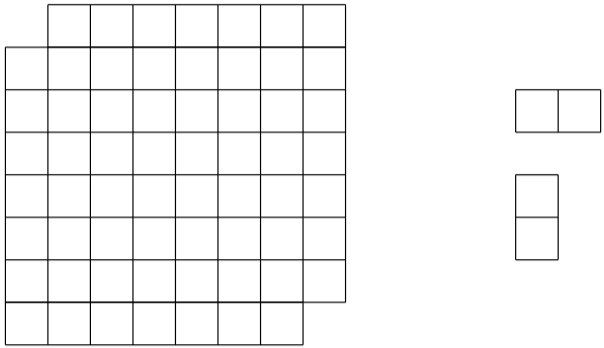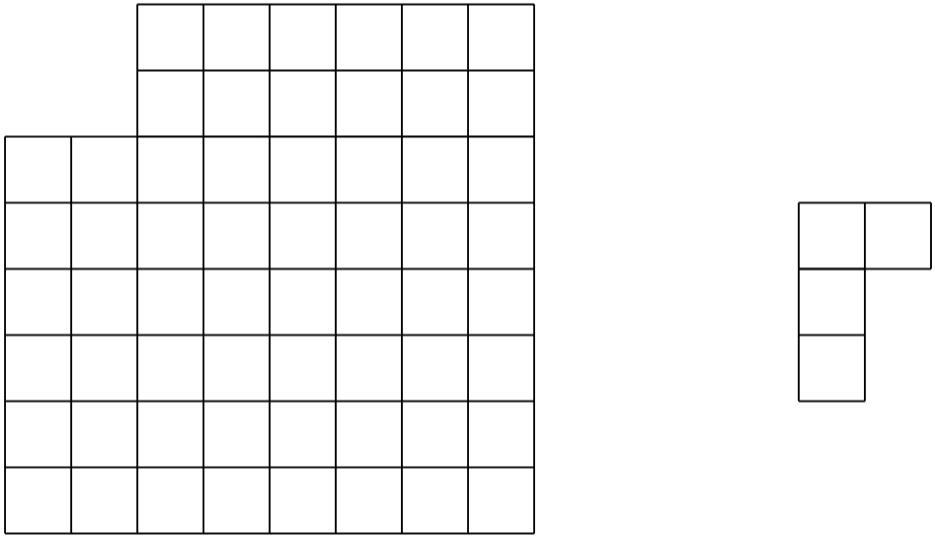###### back to index | new

Colour all the points on a plane either white or black randomly. Show that it is always possible to find a triangle whose vertices have the same colour and its side length is either $1$ or $\sqrt{3}$.

Randomly colour all the points one a plane either black or white. Show that if any two points with a distance of $2$ units have the same colour, then all the points on this plane have the same colour.

$\textbf{Cover the Board}$

Joe cuts off the top left corner and the bottom right corner of an $8\times 8$ board, and then tries to cover the remaining board using thirty-one $1\times 2$ smaller pieces. Is it possible? Note: a smaller piece can be rotated, but cannot be further broken up.$\textbf{Cover the Board (II)}$

Joe cuts off a $2\times 2$ corner from an $8\times 8$ board, and then tries to cover the remaining part using $15$ L-shaped grids made of $4$ grids as shown. Is it possible?Show that among any $6$ people in the world, there must exist $3$ people who either know each other or do not know each other.

There are $6$ points in the $3$-D space. No three points are on the same line and no four points are one the same plane. Hence totally $15$ segments can be created among these points. Show that if each of these $15$ segments is colored either black or white, there must exist a triangle whose sides are of same color.

Seventeen people correspond by mail with one another - each one with all the rest. In their letters only three different topics are discussed. Each pair of correspondents deals with only one of these topics. Prove that there are at least three people who write to each other about the same topic.

Show that it is impossible to cover an $8\times 8$ square using fifteen $4\times 1$ rectangles and one $2\times 2$ square.

Is it possible to arrange these numbers, $1, 1, 2, 2, 3, 3, \cdots, 1986, 1986$ to form a sequence for such there is $1$ number between two $1$'s, $2$ numbers between two $2$'s, $\cdots$, $1986$ numbers between two 1986's?

It is possible to cover a $6\times 6$ grid using one L-shaped piece made of 3 grids and eleven $3\times 1$ smaller grid ?

There are $100$ guests attending a party. If everyone knows at least $67$ other guests, show that there must exist $4$ guests who know each other.

Is it possible to use twenty seven $1\times 2\times 4$ blocks to construct a $6\times 6\times 6$ cube?